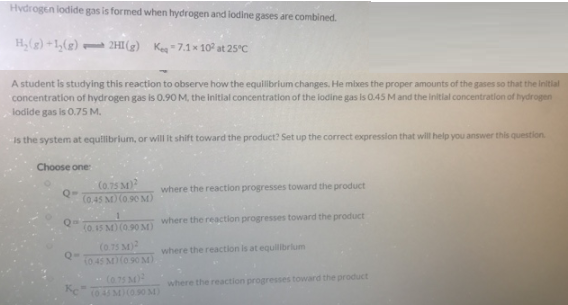# Problem: Hydrogen lodide gas is formed when hydrogen and iodine gases are combined. H2 (g) + I2 (g) ⇌ 2HI (g)     Keq = 7.1 x 102 at 25°CA student is studying this reaction to observe how the equilibrium changes. He mixes the proper amounts of the gases so that the initial concentration of hydrogen gas is 0.90 M, the initial concentration of the lodine gas is 0.45 M and the initial concentration of hydrogen lodide gas is 0.75 M. Is the system at equilibrium, or will it shift toward the product? Set up the correct expression that will help you answer this question.Choose one: (i) Q = (0.75 M)2/(0.45M)(0.90M) where the reaction progresses toward the product (ii) Q = 1/(0.45M)(0.90M) 8 1090 where the reaction progresses toward the product (iii) Q = (0.75 M)2/(0.45M)(0.90M) where the reaction is at equillbrium (iv) KC = (0.75 M)2/(0.45M)(0.90M) where the reaction progresses toward the product

###### FREE Expert Solution
87% (409 ratings)###### Problem Details

Hydrogen lodide gas is formed when hydrogen and iodine gases are combined.

H2 (g) + I2 (g) ⇌ 2HI (g)     Keq = 7.1 x 102 at 25°C

A student is studying this reaction to observe how the equilibrium changes. He mixes the proper amounts of the gases so that the initial concentration of hydrogen gas is 0.90 M, the initial concentration of the lodine gas is 0.45 M and the initial concentration of hydrogen lodide gas is 0.75 M.

Is the system at equilibrium, or will it shift toward the product? Set up the correct expression that will help you answer this question.

Choose one:

(i) Q = (0.75 M)2/(0.45M)(0.90M) where the reaction progresses toward the product

(ii) Q = 1/(0.45M)(0.90M) 8 1090 where the reaction progresses toward the product

(iii) Q = (0.75 M)2/(0.45M)(0.90M) where the reaction is at equillbrium

(iv) KC = (0.75 M)2/(0.45M)(0.90M) where the reaction progresses toward the product